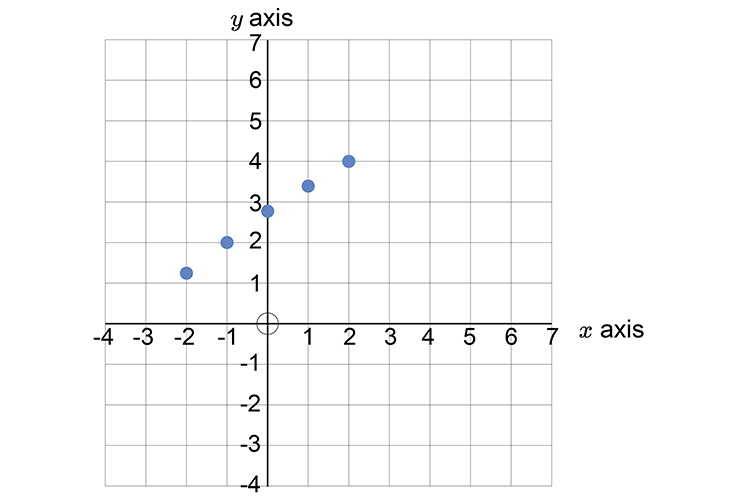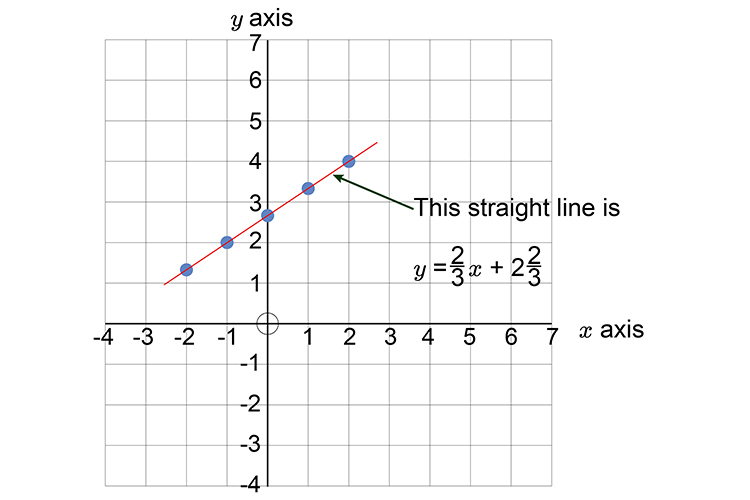# Graphs - Always make y the subject of the formula

The formula for a straight line is y=mx+c

If you are given the formula:

3y-2x=8

Always make y  the subject of the formula.To make y  the subject of the formula follow this procedure:

3y-2x=8

Add 2x  to both sides

3y-2x+2x=8+2x

3y=8+2x

Divide both sides by 3

(3y)/3=(2x+8)/3

Therefore: y=2/3x+8/3

y=2/3x+2\2/3

This line can now be plotted on a graph as follows:

First let's calculate some points on the graph.

If

 x=-2 then y =2/3(-2)+2\2/3 =1.33 x=-1 then y =2/3(-1)+2\2/3 =2 x=0 then y =2/3(0)+2\2/3 =2.66 x=1 then y =2/3(1)+2\2/3 =3.33 x=2 then y =2/3(2)+2\2/3 =4

Now draw the graph and mark out the above points:Now draw a straight line through these points.